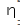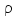Courses

# Bulk Matter - Online Test (12-12-2016)

## 30 Questions MCQ Test | Bulk Matter - Online Test (12-12-2016)

Description
This mock test of Bulk Matter - Online Test (12-12-2016) for Class 11 helps you for every Class 11 entrance exam. This contains 30 Multiple Choice Questions for Class 11 Bulk Matter - Online Test (12-12-2016) (mcq) to study with solutions a complete question bank. The solved questions answers in this Bulk Matter - Online Test (12-12-2016) quiz give you a good mix of easy questions and tough questions. Class 11 students definitely take this Bulk Matter - Online Test (12-12-2016) exercise for a better result in the exam. You can find other Bulk Matter - Online Test (12-12-2016) extra questions, long questions & short questions for Class 11 on EduRev as well by searching above.
QUESTION: 1

Solution:
QUESTION: 2

Solution:
QUESTION: 3

### One litre of a certain fluid weighs 8N. What is its specific volume?

Solution:
QUESTION: 4

When is a liquid said to be not in a boiling or vaporized state?

Solution:
QUESTION: 5

When the angle between surface tension with the liquid (θ) is greater than 90o, the liquid becomes

Solution:
QUESTION: 6

Newton's law of viscosity states that

Solution:
QUESTION: 7

Bernoulli's principle shows that, at points in a moving fluid where potential energy change is very small

Solution:
QUESTION: 8

The location of the centre of pressure over a surface immersed in a liquid is

Solution:
QUESTION: 9

If fluid has constant density then it is said to be

Solution:
QUESTION: 10

According to equation of continuity product of area of pipe and speed of fluid along pipe is

Solution:
QUESTION: 11

Constant of equation of continuity is known as

Solution:
QUESTION: 12

When net force acting on a droplet becomes zero then it falls with

Solution:
QUESTION: 13

A wire of length L and cross section area A is made of a material of Young's modulus Y. If the wire is stretched by an amount x, the work done is

Solution:
QUESTION: 14

For elastomers, stress and strain variation is _________ within elastic limit.

Solution:
QUESTION: 15

The area occupied below the stress-strain graph and above strain axis gives the value of

Solution:
QUESTION: 16

A wire whose cross-section is 4 mm2 is stretched by 0.1 mm by a certain weight. How far will a wire of the same material and length be stretched, if its cross-sectional area is 8 mm2 and the same weight is attached?

Solution:
QUESTION: 17

In Hooke's law, the constant of proportionality signifies

Solution:
QUESTION: 18

The potential energy of a long spring when stretched by 2 cm is U. If the spring is stretched by 8 cm the potential energy stored in it is

Solution:
QUESTION: 19

How does the modulus of elasticity change with temperature?

Solution:
QUESTION: 20

A body is said to be perfectly elastic if

Solution:
QUESTION: 21

Which of the following factors can bring about a change in the elastic properties of a material?

Solution:
QUESTION: 22

The modulus of elasticity of steel is greater than that of rubber because under the same stress

Solution:
QUESTION: 23

A uniform rod of mass m, length L, area of cross-section A and Young's modulus Y hangs from a ceiling. Its elongation under its own weight will be

Solution:
QUESTION: 24

Materials that show very small plastic range beyond elastic limit are called

Solution:
QUESTION: 25

Velocity of efflux flowing through an orifice at height h below the free surface of liquid is given by

Solution:
QUESTION: 26

A wind with speed 40 m/s blows parallel to the roof of a house. The area of the roof is 250 m2. Assuming that the pressure inside the house is atmospheric pressure, the force exerted by the wind on the roof and the direction of the force will be (Pair = 1.2 kg/m3)

Solution:
QUESTION: 27

A flat plate is separated from a large plate by a layer of glycerine of thickness 3 X 10-3m.  If the co-efficient of viscocity of glycerine is 2 Nsm2, what is the force required to keep the plate moving with a velocity of 6 X 10-2 m/s. Area of the plate is 4.8 X 10-3m2.

Solution:
QUESTION: 28

Which of the following assumptions is not considered while arriving at Stoke's law.

Solution:
QUESTION: 29

Find the Reynold's number for the liquid flowing in a pipe of diameter 1.2 cm with average velocity 15 cm/s.  Take= 0.01 poise and= 0.9 gm/cc.

Solution:
QUESTION: 30

A student is performing a test experiment in his laboratory. He takes glycerine, buttermilk and water in three beakers and stirs them thoroughly. He then places the beaker on the table simultaneously. Which of the following will be observed?

Solution: